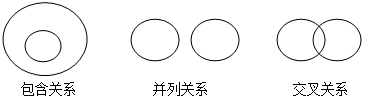QQ:1090930740• 题型：简答题 题类：月考试卷 难易度：简单

年份：2021

通常状况下，酒精${\rm (}$符号${\rm C_{2}H_{5}OH)}$看起来和水外观很像，打开瓶盖会嗅到特殊的香味，取少量酒精点燃后生成能使澄清石灰水变浑浊的气体和水。请根据上述信息回答下列问题：
${\rm (1)}$酒精的性质有 ______ ；
${\rm (2)}$写出酒精燃烧的符号表达式是 ______ 。
• 题型：单选题 题类：月考试卷 难易度：简单

年份：2021

下列生产工艺中，不涉及化学变化的是${\rm (\quad)}$
A、高炉炼铁 B、电解水制氢气 C、海水晒盐 D、煅烧石灰石制生石灰
• 题型：单选题 题类：月考试卷 难易度：简单

年份：2021

下列属于化学变化的是${\rm (\quad)}$
A、电灯通电后发亮 B、从海水中得到食盐 C、稀盐酸除水垢 D、电热棒工作时发热
• 题型：单选题 题类：月考试卷 难易度：中等

年份：2021

人类从自然界获取物质的下列变化中，属于化学变化的是${\rm (\quad)}$
A、工业制氧 B、海水晒盐 C、酿造米酒 D、沙里淘金
• 题型：单选题 题类：月考试卷 难易度：简单

年份：2021

下列变化中不涉及化学变化的是${\rm (\quad)}$
A、钻木取火 B、粮食酿酒 C、活性炭吸附杂质 D、煅烧石灰石
• 题型：单选题 题类：月考试卷 难易度：简单

年份：2021

下列变化中属于物理变化的是${\rm (\quad)}$
A、工业制二氧化碳 B、光合作用 C、活性炭除去冰箱中的异味 D、侯氏制碱
• 题型：单选题 题类：月考试卷 难易度：简单

年份：2021

下列说法正确的是${\rm (\quad)}$
A、“真金不怕火炼”说明金的熔点非常高 B、合金中可以含有非金属 C、“百炼成钢”属于物理变化 D、溶液一定是液体
• 题型：单选题 题类：月考试卷 难易度：简单

年份：2021

物质的用途和性质密切相关。下列用途主要是利用其化学性质的是${\rm (\quad)}$
A、干冰做制冷剂 B、石墨做润滑剂 C、铜丝作导线 D、氢气作高能燃料
• 题型：单选题 题类：其他 难易度：中等

年份：2021

化学概念在逻辑上存在如图所示关系：某化学学习小组收集了以下几组概念，其中属于包含关系的是${\rm (\quad)}$
①饱和溶液与不饱和溶液
②化合物与酸
③物理变化与化学变化
④化合反应与分解反应
⑤缓慢慢氧化与燃烧
⑥酸性溶液和酸溶液
⑦金属材料与合金
A、①④⑥ B、⑤⑦ C、②③ D、②⑥⑦
• 题型：填空题 题类：其他 难易度：一般

年份：2021

在抗击新型冠状病毒肺炎期向做好消毒至关重要，常用的消毒剂有酒精、过氧乙酸和“${\rm 84}$”消毒液等。
${\rm (1)}$医用酒精是${\rm 75％}$酒精溶液，其溶剂是 ______ 。
${\rm (2)}$过氧乙酸具有强氧化性，能让病毒表面的蛋白质外壳变质，致使病毒死亡。强氧化性属于 ______ ${\rm (}$填物理或者化学${\rm )}$性质。若将溶质质量分数为${\rm 0.5％}$的过氧乙酸溶液${\rm 1000g}$和质量分数为${\rm 14％}$的过氧乙酸溶液${\rm 500g}$混合可配制溶质质量分数为 ______ 的溶液，该溶液可以用 ______ 填${\rm (}$“塑料桶”或“铁桶”${\rm )}$盛装。
${\rm (3)}$“${\rm 84}$”消毒液的有效成分是次氯酸钠${\rm (NaClO)}$属于 ______ ${\rm (}$填序号${\rm )}$。
A.酸
B.碱
C.盐
D.氧化物
E.含氧化合物